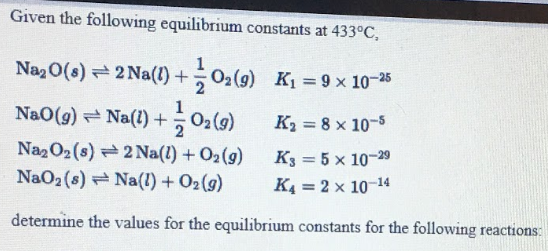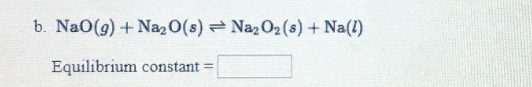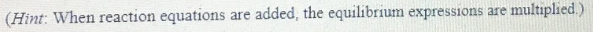# Given the following equilibrium constants at 433 degrees C, determine the values for the equilibrium constants for the following reactions: b) NaO(g) + Na2O(s) ⇌ Na2O2(s) + Na(l) (Hint: When reaction equations are added, the equilibrium expressions are multiplied.)